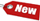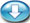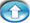Browse Accounting Lessons HereAccounting Terms & DefinitionsAccounting for Merchandising ActivitiesDebits and Credits (Double Entry Accounting)Business Valuation FormulasTime Value of Money & Present/Future ValuesComplex Debt & Equity InstrumentsCommon Stock & Shareholder's EquityAccounting & Finance RatiosValuing Common StockCorporate Income TaxesLower of Cost or Market (LCM) & Inventory ValuationChart of Accounts & BookkeepingBonds Payable & Long Term LiabilitiesCapital AssetsGAAP, Accrual & Cash Accounting, Information Commodity, Internal Controls & MaterialityWhat category of browser are you on this website? Accounting student (homework help) Finance professor (university research) Accounting manager (at work) Other Explore Careers in Accounting and FinanceVisit our section on Careers in Accounting & Finance to explore vast opportunities in this industry.

Chapter 4.4® - Changing Advanced Function Keys (BGN, C/Y, P/Y), Converting from Nominal Interest to Effective Interest Rates using BAII Financial Calculator

In some advanced calculations, you might have 4 periods in a year (quarterly) or just 2 periods in a year (semi-annual) or even 12 periods in a year (monthly). So far we have assumed our calculation above on 1 period a year (annual). Other calculations might require you to set monthly compounding instead of annual compounding. How do you deal with these changes?

Compounding of Interest per Period (C/Y) and Number of Periods per Year (P/Y)

Example 1: Say there are 4 periods per year, and monthly compounding. Do the following in your calculator:

 i) Press 2nd I/Y ii) P/Y = 4, press Enter theniii) C/Y = 12, press Enter, theniv) Once these entries have been made, exit from this area by pressing CE/C.

Interest Rate Conversion using BA II Plus Financial Calculator

This function is used for finding nominal and effective rates of interest, that are slightly different from each other.

 NOM = Nominal rate of interest (stated rate of interest). EFF = Effective rate of interest (annual percentage rate).

Example: If 8% is compounded monthly, what is the effective rate of interest?

 i) Press 2nd, and then press 2 ii) NOM = Press 8, Enter theniii) EFF= This is the variable that needs to be calculated, so pressto bypass it for now. iv) C/Y = Press 12, then Enter, thenv) EFF = Press CPT vi) Final Answer = 8.3%

Exercise Problems for Converting Nominal/Effective Interest Rates

1) Calculate the ANNUAL effective rate given a nominal interest rate of 8%

 On your BA II plus financial calculator i) Press 2nd, and then press 2 ii) NOM = Press 8, Enter theniii) EFF= Pressiv) C/Y = Press 1, then Enter, thenv) EFF = Press CPT vi) Answer = 8%

2) Calculate the SEMI-ANNUAL effective rate given a nominal interest rate of 8%

 On your BA II plus financial calculator i) Press 2nd, and then press 2 ii) NOM = Press 8, Enter theniii) EFF= Pressiv) C/Y = Press 2, then Enter, thenv) EFF = Press CPT vi) Answer = 8.16%

3) Calculate the QUARTERLY effective rate given a nominal interest rate of 8%

 On your BA II plus financial calculator i) Press 2nd, and then press 2 ii) NOM = Press 8, Enter theniii) EFF= Pressiv) C/Y = Press 4, then Enter, thenv) EFF = Press CPT vi) Answer = 8.24%

4) Calculate the MONTHLY effective rate given a nominal interest rate of 8%

 On your BA II plus financial calculator i) Press 2nd, and then press 2 ii) NOM = Press 8, Enter theniii) EFF= Pressiv) C/Y = Press 12, then Enter, thenv) EFF = Press CPT vi) Answer = 8.299% or round up to 8.3%